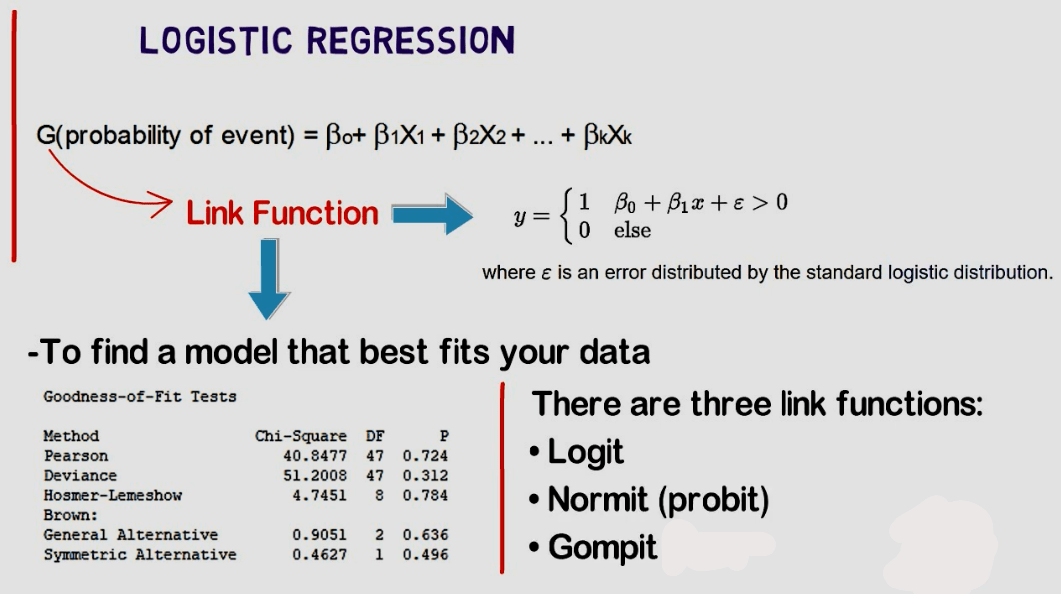#.Image Source: Statistical Aid
Logistic regression is a statistical technique to find the association between the categorical dependent (response) variable and one or more categorical or continuous independent (explanatory) variable.
We can define the regression model as,

G(probability of event)=β01x12x2+…+βkxk

We determine G using link function as following,

Y={1 ; β01x1+ϵ>0

{0 ; else

There are three types of link fuction. They are,

• Logit
• Normit (probit)
• Gombit## Why we use logistic regression?

We use it when there exists,

• One Categorical response variable
• One or more explanatory variable.
• No linear relationship between dependent and independent variables.

## Assumptions of Logistic Regression

• The dependent variable should be categorical (binary, ordinal, nominal or count occurrences).
• The predictor or independent variable should be continuous or categorical.
• The correlation among the predictors or independent variable (multi-collinearity) should not be severe but there exists linearity of independent variables and log odds.
• The data should be the representative part of population and record the data in the order its collected.
• The model should provide a good fit of the data.

## Logistic regression vs Linear regression

• In the case of Linear Regression, the outcome is continuous while in the case of logistic regression outcome is discrete (not continuous)
• To perform linear regression, we require a linear relationship between the dependent and independent variables. But to perform Logit we do not require a linear relationship between the dependent and independent variables.
• Linear Regression is all about fitting a straight line in the data while Logit  is about fitting a curve to the data.
• Linear Regression is a regression algorithm for Machine Learning while Logit  is a classification Algorithm for machine learning.
• Linear regression assumes Gaussian (or normal) distribution of the dependent variable. Logit assumes the binomial distribution of the dependent variable.

*Logit=logistic regression

## Types

There are four types of logistic regression. They are,

• Binary logistic: When the dependent variable has two categories and the characteristics are at two levels such as yes or no, pass or fail, high or low etc. then the regression is called binary logistic regression.
• Ordinal logistic: When the dependent variable has three categories and the characteristics are at natural ordering of the levels such as survey results (disagree, neutral, agree) then the regression is called ordinal logistic regression.
• Nominal logistic: When the dependent variable has three or more categories but the characteristics are not at natural ordering of the levels such as colors (red, blue, green) then the regression is called nominal logistic.
• Poisson logistic: When the dependent variable has three or more categories but the characteristics are the number of time of an event occurs such as 0, 1, 2, 3, …, etc.  then the regression is called Poisson logistic regression.

Source...

Views: 1648

Comment